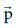Home | | Physics 11th std | Relation between Momentum and Kinetic Energy

# Relation between Momentum and Kinetic Energy

Relation between Momentum and Kinetic Energy

Relation between Momentum and Kinetic Energy

Consider an object of mass m moving with a velocity. Then its linear momentum isand its kinetic energy, KE =  ½ mv2 .Multiplying both the numerator and denominator of equation (4.13) by mass, mwhereis the magnitude of the momentum. The magnitude of the linear momentum can be obtained byNote that if kinetic energy and mass are given, only the magnitude of the momentum can be calculated but not the direction of momentum. It is because the kinetic energy and mass are scalars.

Study Material, Lecturing Notes, Assignment, Reference, Wiki description explanation, brief detail
11th Physics : UNIT 4 : Work, Energy and Power : Relation between Momentum and Kinetic Energy |